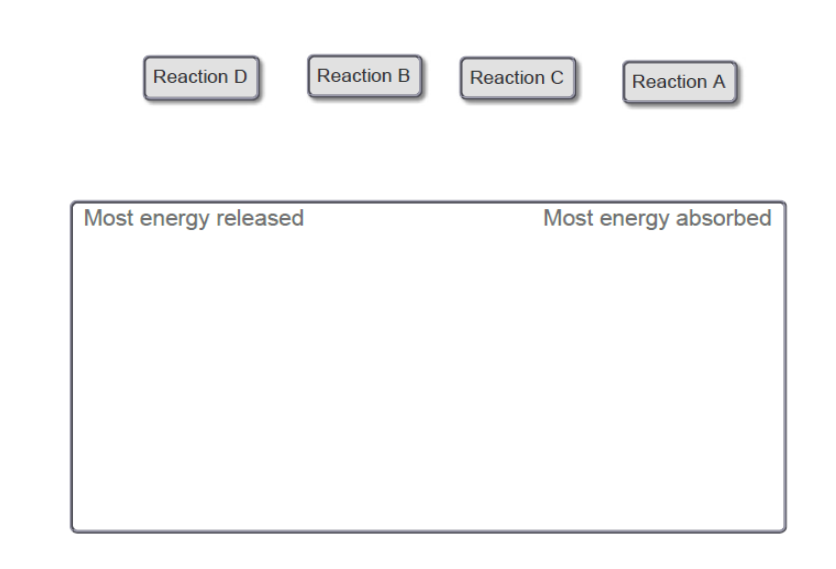# Problem: Assuming constant pressure, rank these reactions from most energy released by the system to most energy absorbed by the system, based on the following descriptions:A. Surroundings get colder and the system decreases in volume.B. Surroundings get hotter and the system expands in volume.C. Surroundings get hotter and the system decreases in volume.D. Surroundings get hotter and the system does not change in volume.Also assume that the magnitude of the volume and temperature changes are similar among the reactions.Rank from most energy released to most energy absorbed. To rank items as equivalent, overlap them.Energy cannot be created nor destroyed, but it can be transferred between a reaction and its surroundings. The change in energy, ΔE, is positive if the reaction absorbs energy, and it is negative if the reaction releases energy. You may also see this expressed in terms of change in internal energy, ΔU. For the purposes of this question, ΔU and ΔE are equal.One way a reaction can transfer energy to or from the surroundings is by releasing or absorbing heat. A reaction can also transfer energy, in the form of work, through a change in volume. The total change in energy is the sum of the heat and work:ΔE=q+wAt constant pressure, q = ΔH and w = −PΔV and soΔE=ΔH-PΔV

###### FREE Expert SolutionView Complete Written Solution
###### Problem Details

Assuming constant pressure, rank these reactions from most energy released by the system to most energy absorbed by the system, based on the following descriptions:

A. Surroundings get colder and the system decreases in volume.
B. Surroundings get hotter and the system expands in volume.
C. Surroundings get hotter and the system decreases in volume.
D. Surroundings get hotter and the system does not change in volume.

Also assume that the magnitude of the volume and temperature changes are similar among the reactions.

Rank from most energy released to most energy absorbed. To rank items as equivalent, overlap them.Energy cannot be created nor destroyed, but it can be transferred between a reaction and its surroundings. The change in energy, ΔE, is positive if the reaction absorbs energy, and it is negative if the reaction releases energy. You may also see this expressed in terms of change in internal energy, ΔU. For the purposes of this question, ΔU and ΔE are equal.

One way a reaction can transfer energy to or from the surroundings is by releasing or absorbing heat. A reaction can also transfer energy, in the form of work, through a change in volume. The total change in energy is the sum of the heat and work:

$\mathbf{\Delta E}\mathbf{=}\mathbf{q}\mathbf{+}\mathbf{w}$

At constant pressure, q = ΔH and w = −PΔV and so

$\mathbf{\Delta E}\mathbf{=}\mathbf{\Delta H}\mathbf{-}\mathbf{P\Delta V}$